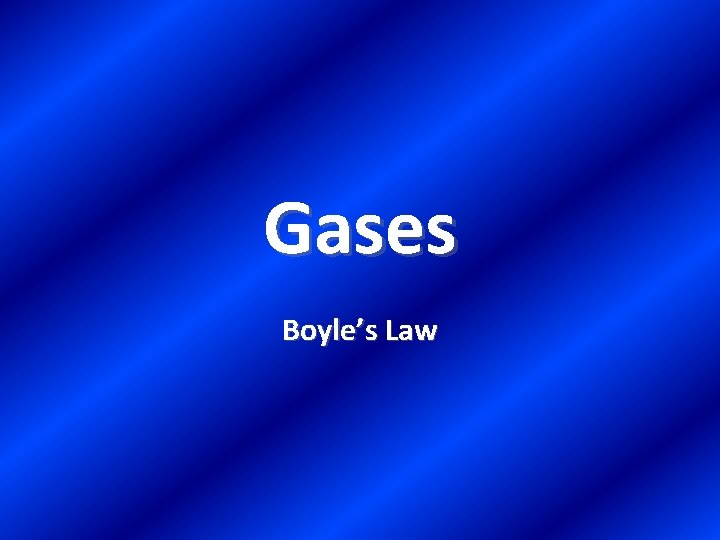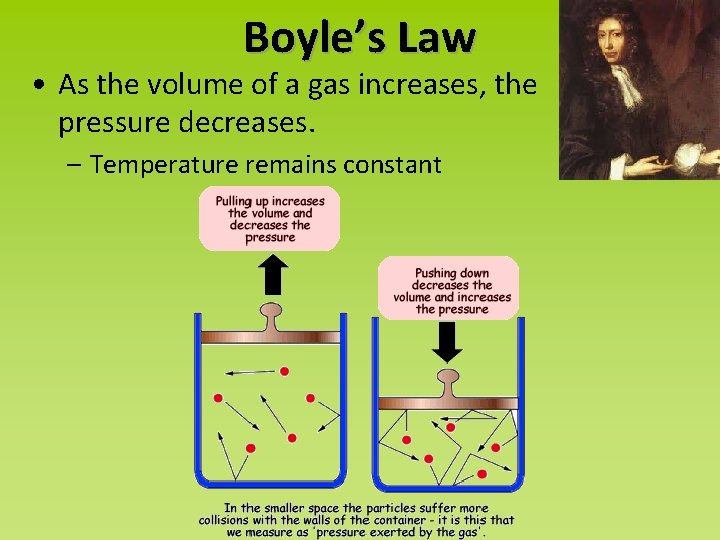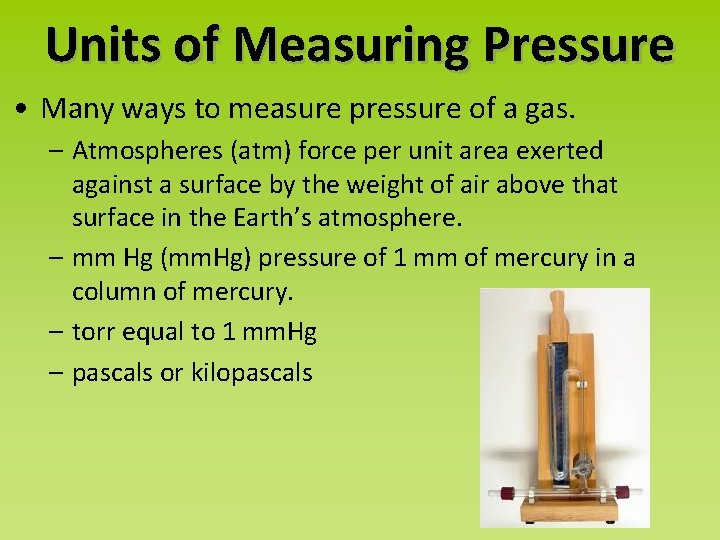# Gases Boyles Law Boyles Law As the volume

• Slides: 6Gases Boyle’s LawBoyle’s Law • As the volume of a gas increases, the pressure decreases. – Temperature remains constantSymbolic Representation of Boyle’s Law As volume increases, pressure decreases Pressure and temperature are inversely proportional Pressure (atm) Volume (L) How is this represented in the form of an P V 1 1 equation? = P 2 V 2Units of Measuring Pressure • Many ways to measure pressure of a gas. – Atmospheres (atm) force per unit area exerted against a surface by the weight of air above that surface in the Earth’s atmosphere. – mm Hg (mm. Hg) pressure of 1 mm of mercury in a column of mercury. – torr equal to 1 mm. Hg – pascals or kilopascalsBoyle’s Law Example #1 A balloon contains 20. 0 L of helium gas at 1. 02 atm. The balloon rises to an altitude where the pressure is only 0. 15 atm. What is the volume of the balloon at the higher altitude? Step #1: Qualitative Assessment of the Problem Step #2: Quantitative Assessment of the ProblemBoyle’s Law Example #2 A gas with a volume of 4. 00 L at a pressure of 2. 02 atm is allowed to expand to a volume of 12. 0 L. What is the pressure in the container if the temperature remains constant? Step #1: Qualitative Assessment of the Problem Step #2: Quantitative Assessment of the Problem